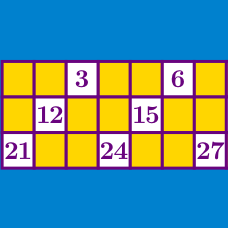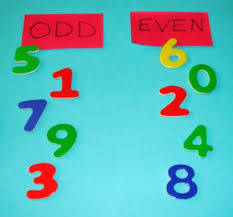Number Theory

# Divisibility: Level 2 Challenges

Is 87985 divisible by 7?

Find the smallest positive multiple of nine containing only even digits.

How many perfect squares have the property that all of their digits are equal?

Note: $0=0^2$ is a perfect square.Given that $a$ and $b$ are integers, what can we conclude about the expression

$\big( { a }^{ 2 }+a+2011 \big) ( 2b+1 ) ?$

$\frac{(n+2)!}{(n-1)!}$ The above fraction is divisible by which of the following?

×

Problem Loading...

Note Loading...

Set Loading...